## TI-Nspire™ CX II and CX II CAS graphing calculator specifications

 General Screen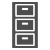Storage 90+ MB storage memory 64 MB operating memory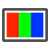Color Backlit display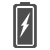Power TI Rechargeable Battery (included) USB port for computer connectivity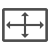Size 320 x 240 pixels (3.2" diagonal)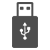Connector Standard A to Mini-B USB cable included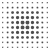Resolution 125 DPI; 16-bit color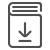Guidebook TI-Nspire™ guidebooks are available to help you learn to use TI-Nspire™ technology.Download

#### Built-in functionality

• Function, parametric, polar, sequence and 3D graphing
• Multiple graphing functions can be defined, saved, graphed and analyzed at one time
• User-defined list names in Lists & Spreadsheet
• Eleven interactive zoom features
• Numeric evaluations in table format for all graphing modes
• Interactive analysis of function values, roots, maximums, minimums, integrals and derivatives
• Six different graph styles and 15 colors to select from for differentiating the look of each graph drawn
• Matrix operations: transpose and augment matrices; use elementary row operations; find inverse, determinant and reduced row echelon forms; convert matrices to lists and vice versa; calculate eigenvalues and eigenvectors
• List-based one- and two-variable statistical analysis, including logistic, sinusoidal, median-median, linear, logarithmic, exponential, power, quadratic polynomial, cubic polynomial, and quartic polynomial regression models
• Three statistical plot definitions for scatter plots, xy-line plots, histograms, regular and modified box-and-whisker plots, and normal probability plots
• Advanced statistics analysis, including 10 hypothesis testing functions, seven confidence interval functions and one-way analysis of variance
• Eighteen probability distributions functions, including the cumulative distribution function (CDF), probability density function (PDF) and inverse probability distribution function for normal, chi-squared, t-, and F- distributions; and the CDF and PDF for binomial, geometric and Poisson distributions
• Built-in interactive geometry capabilities
• Quick alpha keys
• Simple drop-down menus emulate the intuitive operation of familiar computer features
• TI-Nspire™ Documents (.tns files) can be created, edited, saved and reviewed
• Use images (.jpeg, .jpg, .bmp, .png formats) that can be overlaid with graphical elements
• Explore mathematical expressions in symbolic form, see patterns and understand the math behind the formulas
• Visualize multiple representations of a single problem-algebraic, graphical, geometric, numeric and written
• Chem Box feature allows easy input of chemical formulas and equations
• Manipulate linked representations of properties to instantly update the others and show meaningful connections without switching screens (for example, grab a graphed function and move it to see the effect on corresponding equations and data lists)
TI-Nspire™ CX II CAS functionality
• Computer Algebra System (CAS) capabilities
• CAS can be disabled

#### Programming

• Different options for coding languages including Python and TI-Basic.
• Features a dedicated programming environment as well as programming libraries for global access to user-defined functions and programs.

#### Connectivity

• Electronically upgradeable graphing handheld allows you to have the most up-to-date functionality
• Standard A to Mini-B USB cable included for computer connectivity and charging

#### Data-collection support

• Compatible with TI-Nspire™ Lab Cradle, Vernier EasyLink® USB Sensor and Vernier EasyTemp® USB temperature sensor systems to allow collection and analysis of real-world data

#### Accessories

• TI Rechargeable Battery
• TI-Nspire™ Docking Station